### Home > CAAC > Chapter 14 > Lesson 14.3.1.6 > Problem3-79

3-79.
1. Solve each equation below. Homework Help ✎

1. 2(x−1) = 64

2. 4.7 = x1/3

3. 8(x+3) = 16x

4. 93 = 27(2x−1)

5. x6 = 29

6. 25x = 125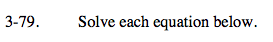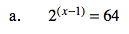64 = 26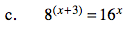Look for a common base.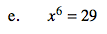x ≈ ± 1.75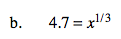Try cubing both sides.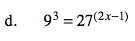See part (c).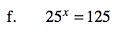See part (c).